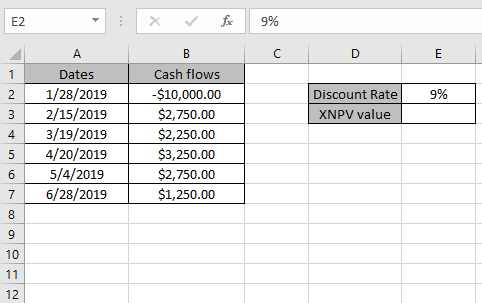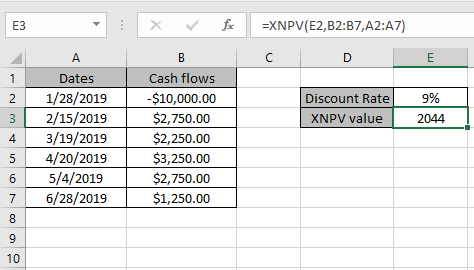# How to use the XNPV function in Excel

In simple words, to get the net present value of a series of cash flows at irregular intervals.

XNPV function returns the net present value for a schedule of cash flows that is not necessarily periodic
Syntax:

=XNPV (rate, values, dates)

Rate : discount rate
Values : Cash flows
Dates : Dates that corresponds to cash flows
Let’s understand this function using it in an example.Here we have a list of Cash flows with corresponding dates. Discount rate is 9%.

Use the formula:

=XNPV(E2, B2:B7, A2:A7)

E2 : Discount rate
B2:B7 : Cash flows
A2:A7 : Dates that corresponds to cash flowsThe function returns the net present value for the Cash flow data shown in the above snapshot.
Notes:

1. Dates should be valid date in Excel
2. Dates and Cash flows must be in order.
3. The function doesn’t count the initial cash flow.
4. To calculate the net present value for a series of cash flows that is periodic, use the NPV function.

Hope you understood How to use the XNPV function in Excel. Explore more articles on Excel financial functions here. Please state your query or feedback in the comment box below.

Related Articles:

How to use the Excel IPMT Function

How to Use Compound Interest Function in Excel

Popular Articles:

50 Excel Shortcuts to Increase Your Productivity

How to use the VLOOKUP Function in Excel

How to use the COUNTIF in Excel 2016

How to use the SUMIF Function in Excel

Terms and Conditions of use

The applications/code on this site are distributed as is and without warranties or liability. In no event shall the owner of the copyrights, or the authors of the applications/code be liable for any loss of profit, any problems or any damage resulting from the use or evaluation of the applications/code.